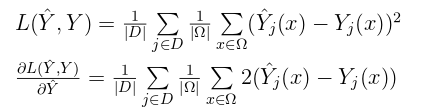# Using own loss while having gradients and numpy implementation

Dear all,

I would like to implement my own loss in pytorch.

There are two things I would like to use:

1. numpy implementation of a loss
2. numpy implementation of the derivative of my loss with respect to inputs.

Is that possible?

Here is an example: lets say I would like to implement a simple MSE between two
matrices, predictions Yhat and targets Y, of shape batch_sizex32x32x32:where j goes over training examples in our batch D, and x goes over every
value of the 32x32x32 grid

I can quickly implement it in numpy:

``````def my_mse_np(predictions, targets):
loss_np = np.mean(np.mean((X.reshape(batch_size,-1) - Y.reshape(batch_size,-1))**2, axis=1))
return loss_np
``````

and now, I also calculated the gradient (see image above)

and implemented it in numpy:

``````def my_mse_grad_np(predictions, targets)
grad_np = 2*np.mean(np.mean(X.reshape(batch_size,-1) - Y.reshape(batch_size,-1), axis=1))